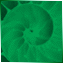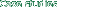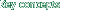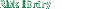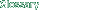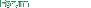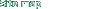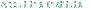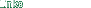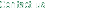Risk Library
 Documents by Author
 Committees at the Bank for International...
 Amendment of the Capital Accord to Incor...
 Part B - Use of internal models to measu...B.3 Specification of Market Risk Factors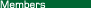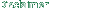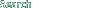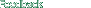### B.3 Specification of Market Risk Factors

 An important part of a bank's internal market risk measurement system is the specification of an appropriate set of market risk factors, i.e., the market rates and prices that affect the value of the bank's trading positions. The risk factors contained in a market risk measurement system should be sufficient to capture the risks inherent in the bank's portfolio of on- and off-balance sheet trading positions. Although banks will have some discretion in specifying the risk factors for their internal models, the following guidelines should be fulfilled. For interest rates, there must be a set of risk factors corresponding to interest rates in each currency in which the bank has interest-rate-sensitive on- or off-balance sheet positions. The risk measurement system should model the yield curve using one of a number of generally accepted approaches, for example, by estimating forward rates of zero coupon yields. The yield curve should be divided into various maturity segments in order to capture variation in the volatility of rates along the yield curve; there will typically be one risk factor corresponding to each maturity segment. For material exposures to interest rate movements in the major currencies and markets, banks must model the yield curve using a minimum of six risk factors. However, the number of risk factors used should ultimately be driven by the nature of the bank's trading strategies. For instance, a bank with a portfolio of various types of securities across many points of the yield curve and that engages in complex arbitrage strategies would require a greater number of risk factors to capture interest rate risk accurately. The risk measurement system must incorporate separate risk factors to capture spread risk (e.g., between bonds and swaps). A variety of approaches may be used to capture the spread risk arising from less than perfectly correlated movements between government and other fixed-income interest rates, such as specifying a completely separate yield curve for non-government fixed-income instruments (for instance, swaps or municipal securities) or estimating the spread over government rates at various points along the yield curve. For exchange rates (which may include gold), the risk measurement system should incorporate risk factors corresponding to the individual foreign currencies in which the bank's positions are denominated. Since the value-at-risk figure calculated by the risk measurement system will be expressed in the bank's domestic currency, any net position denominated in a foreign currency will introduce a foreign exchange risk. Thus, there must be risk factors corresponding to the exchange rate between the domestic currency and each foreign currency in which the bank has a significant exposure. For equity prices, there should be risk factors corresponding to each of the equity markets in which the bank holds significant positions: at a minimum, there should be a risk factor that is designed to capture market-wide movements in equity prices (e.g., a market index). Positions in individual securities or in sector indices could be expressed in "beta-equivalents" relative to this market-wide index; a somewhat more detailed approach would be to have risk factors corresponding to various sectors of the overall equity market (for instance, industry sectors or cyclical and non-cyclical sectors). As above, positions in individual stocks within each sector could be expressed in beta-equivalents49 relative to the sector index; the most extensive approach would be to have risk factors corresponding to the volatility of individual equity issues. The sophistication and nature of the modelling technique for a given market should correspond to the bank's exposure to the overall market as well as its concentration in individual equity issues in that market. For commodity prices, there should be risk factors corresponding to each of the commodity markets in which the bank holds significant positions (also see paragraph 6 of A.4): for banks with relatively limited positions in commodity-based instruments, a straightforward specification of risk factors would be acceptable. Such a specification would likely entail one risk factor for each commodity price to which the bank is exposed. In cases where the aggregate positions are quite small, it might be acceptable to use a single risk factor for a relatively broad sub-category of commodities (for instance, a single risk factor for all types of oil); for more active trading, the model must also take account of variation in the "convenience yield" between derivatives positions such as forwards and swaps and cash positions in the commodity.